﻿ 还没搞懂C语言指针？一文详解C指针

### 还没搞懂C语言指针？一文详解C指针

2021-12-2 14:38:53

hello 你好呀!,我是aoz,今天我们来一起研究c指针这个东西

### 文章目录

• 1. 为什么需要指针？
• 2. 指针的样子
• 3. 内存与变量
• 4. 指针变量和指向关系
• 5. 定义指针变量
• 6. 指针的2个重要属性
• 7. 取地址
• 8. 解引用
• 9. 指针之间的赋值
• 10. 空指针（NULL指针）
• 11. 坏指针
• 12. void\*类型指针
• 13. 结构体和指针
• 14. 数组和指针
• 15. 函数和指针
• 函数的参数和指针
• 函数的指针
• 16. const和指针
• 17. 深拷贝和浅拷贝
• 18. 附加知识

## 1. 为什么需要指针？

👆👆👆 这三点就是指针存在的意义,最后一条则是实际使用的一点心得(仅对新手有用)

## 2. 指针的样子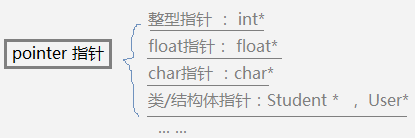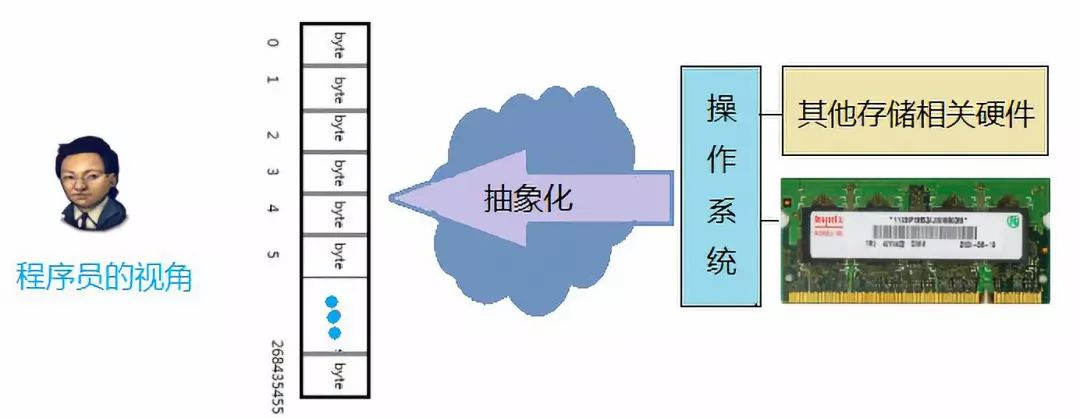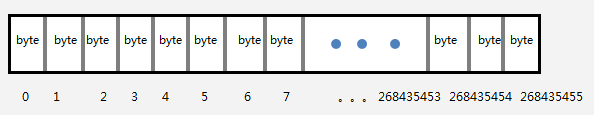👆👆👆上面一堆废话就是告诉你内存是啥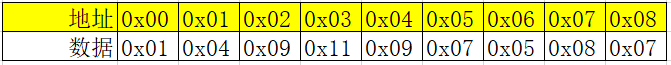``````	unsigned char * a = 0x00; //定义了一个指向0x00的指针
``````

``````	unsigned int * a = 0x00; //定义了一个指向0x00的指针
``````

==========================================================================================================================

## 3. 内存与变量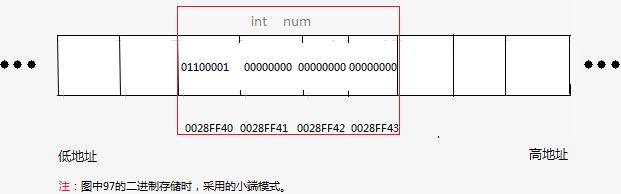1、内存的数据

97的二进制是 : 00000000 00000000 00000000 0110000 , 但使用的小端模式存储时，低位数据存放在低地址，所以图中画的时候是倒过来的。

2、内存数据的类型

num的类型是int，因此将被解释为 一个整数。

3、内存数据的名称

4、内存数据的地址

5、内存数据的生命周期

num是main函数中的局部变量，因此当main函数被启动时，它被分配于栈内存上，当main执行结束时，消亡。

C语言中的程序数据会按照他们定义的位置，数据的种类，修饰的关键字等因素，决定他们的生命周期特性。

## 4. 指针变量和指向关系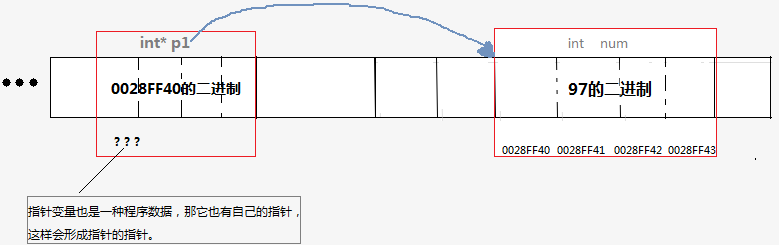## 5. 定义指针变量

C语言中，定义变量时，在变量名前写一个 * 星号，这个变量就变成了对应变量类型的指针变量。必要时要加( ) 来避免优先级的问题。

``````int a ; //int类型变量 a
int *a ; //int* 变量a
int arr; //arr是包含3个int元素的数组
int (* arr ); //arr是一个指向包含3个int元素的数组的指针变量

//-----------------各种类型的指针------------------------------
int* p_int; //指向int类型变量的指针
double* p_double; //指向idouble类型变量的指针
struct Student *p_struct; //结构体类型的指针
int(*p_func)(int,int); //指向返回类型为int，有2个int形参的函数的指针
int(*p_arr); //指向含有3个int元素的数组的指针
int** p_pointer; //指向 一个整形变量指针的指针
``````

## 6. 指针的2个重要属性

``````int main(void)
{
int num = 97;
int *p1  = &num;
char* p2 = (char*)(&num);
printf("%d",*p1);    //输出  97
putchar(*p2);          //输出  a
return 0;
}
``````

*p1 : 将从地址0028FF40 开始解析，因为p1是int类型指针，int占4字节，因此向后连续取4个字节，并将这4个字节的二进制数据解析为一个整数 97。

*p2 : 将从地址0028FF40 开始解析，因为p2是char类型指针，char占1字节，因此向后连续取1个字节，并将这1个字节的二进制数据解析为一个字符，即’a’。

## 7. 取地址

``````int add(int a , int b)
{
return a + b;
}

int main(void){
int num = 97;
float score = 10.00F;
int arr = {1,2,3};
//-----------------------
int* p_num = &num;
float* p_score = &score;
int (*p_arr) = &arr;
return 0;
}
``````

• 数组名的值就是这个数组的第一个元素的地址。
• 函数名的值就是这个函数的地址。
• 字符串字面值常量作为右值时，就是这个字符串对应的字符数组的名称,也就是这个字符串在内存中的地址。
``````int add(int a , int b){
return a + b;
}

int main(void)
{
int arr = {1,2,3};
//-----------------------
int* p_first = arr;
const char* msg = "Hello world";
return 0;
}
``````

## 8. 解引用

``````int main(void)
{
int age = 19;
int*p_age = &age;
*p_age  = 20;  //通过指针修改指向的内存数据
printf("age = %d",*p_age);   //通过指针读取指向的内存数据
printf("age = %d",age);
return 0;
}
``````

## 9. 指针之间的赋值

``````int* p1  = & num;int* p3 = p1;
//通过指针 p1 、 p3 都可以对内存数据 num 进行读写，如果2个函数分别使用了p1 和p3，那么这2个函数就共享了数据num。
``````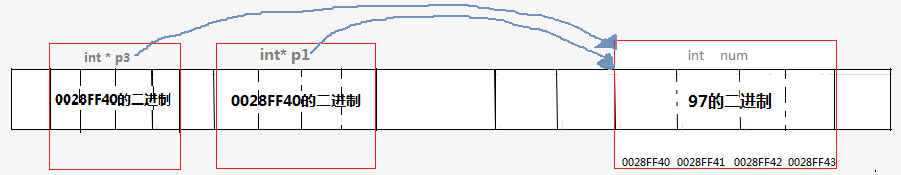## 10. 空指针（NULL指针）

NULL是C语言标准定义的一个值，这个值其实就是0，只不过为了使得看起来更加具有意义，才定义了这样的一个宏，中文的意思是空，表明不指向任何东西。你懂得。不过在此不讨论空和零的区别。

``````#ifdef __cplusplus
#define NULL    0
#else
#define NULL   ((void *)0)
#endif
``````

NULL指针的概念非常有用，它给了你一种方法，表示某个特定的指针目前并未指向任何东西。例如，一个用于在某个数组中查找某个特定值的函数可能返回一个指向查找到的数组元素的指针。如果没找到，则返回一个NULL指针。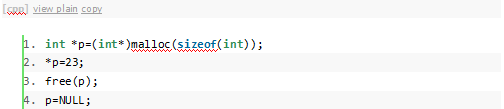free函数是不会也不可能把p置空的。像下面这样的代码就会出现内存段错误：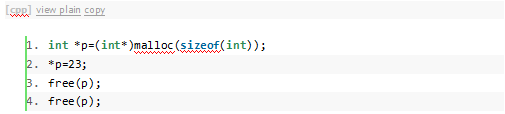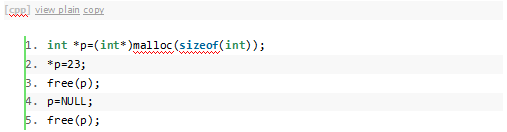**这里顺便告诉大家一个内存释放的小窍门，可以有效的避免因为忘记对指针进行置空而出现各种内存问题。****这个方法就是自定义一个内存释放函数，但是传入的参数不知指针，而是指针的地址，在这个函数里面置空，如下：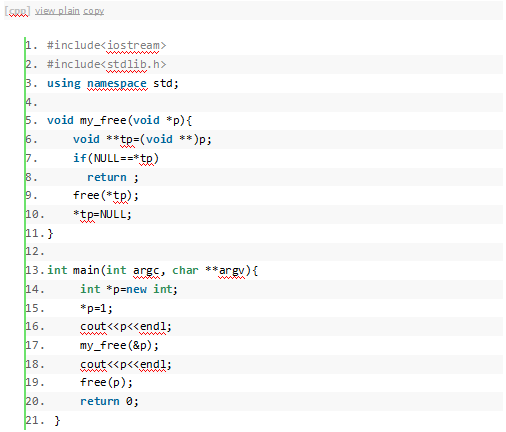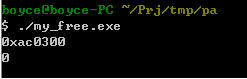my_free调用了之后，p的值就变成了0（NULL），调用多少次free都不会报错了！

**另外一个方式也非常有效，那就是定义FREE宏，在宏里面对他进行置空。**例如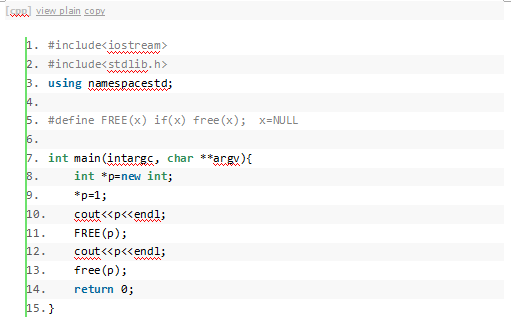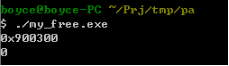（关于内存的动态分配，这是个比较复杂的话题，有机会再专门开辟一章给各位讲述一下吧，写个帖子还是很花费时间和精力的，呵呵，写过的童鞋应该都很清楚，所以顺便插一句，转帖可以，请注明出处，毕竟，大家都是本着共享的精神来讨论问题的，写的好坏都没有向你所要什么，请尊重每个人的劳动成果。）

## 11. 坏指针

``````下面的代码就是错误的示例。

void opp()
{
int*p = NULL;
*p = 10;      //Oops! 不能对NULL解地址
}
void foo()
{
int*p;
*p = 10;      //Oops! 不能对一个未知的地址解地址
}
void bar()
{
int*p = (int*)1000;
*p =10;      //Oops!   不能对一个可能不属于本程序的内存的地址的指针解地址
}
``````

## 12. void*类型指针

void指针实际上的意思是指向任意类型的指针。任意类型的指针都可以直接赋给void指针，而不需要进行强制转换。

``````Type a, *p=&a;（Type等于char, int, struct, int *…）void *pv;
pv=p;
``````

``````void free(void*ptr);
``````

free函数的参数可以是任意指针，没有谁见过free参数里面的指针需要强壮为void*的吧？

malloc, calloc,realloc这些函数的返回值也是void指针，因为内存分配，实际上只需要知道分配的大小，然后返回新分配内存的地址就可以了，指针的值就是地址，返回的不管是何种指针，其实结果都是一样的，因为所有的指针长度其实都是32位的（32位机器），它的值就是内存的地址，指针类型只是给编译器看的，目的是让编译器在编译的时候能够正确的设置指针的值（参见指针运算章节）。如果malloc函数设置成下面这样的原型，完全没有问题。

``````char*malloc(size_t sz);
``````

``````Type* malloc(size_t sz);
``````

## 13. 结构体和指针

``````typedef struct{
char name;
int age;
float score;
}
Student;

int main(void)
{
Student stu = {"Bob" , 19, 98.0};
Student*ps = &stu;
ps->age = 20;
ps->score = 99.0;
printf("name:%s age:%d",ps->name,ps->age);
return 0;
}
``````

## 14. 数组和指针

1、数组名作为右值的时候，就是第一个元素的地址。

``````int main(void){
int arr = {1,2,3};
int*p_first = arr;
printf("%d",*p_first);  //1
return 0;
}
``````

2、指向数组元素的指针 支持 递增 递减 运算。（实质上所有指针都支持递增递减 运算 ，但只有在数组中使用才是有意义的）

``````int main(void){
int arr = {1,2,3};
int*p = arr;
for(;p!=arr+3;p++)
{
printf("%d",*p);
}
return 0;
}
``````

3、p= p+1 意思是，让p指向原来指向的内存块的下一个相邻的相同类型的内存块。

4、p[n] == *(p+n)

p[n][m] == *( *(p+n)+ m )

5、当对数组名使用sizeof时，返回的是整个数组占用的内存字节数。当把数组名赋值给一个指针后，再对指针使用sizeof运算符，返回的是指针的大小。

``````int main(void)
{
int arr = {1,2,3};
int*p = arr;
printf("sizeof(arr)=%d",sizeof(arr));  //sizeof(arr)=12
printf("sizeof(p)=%d",sizeof(p));   //sizeof(p)=4
return 0;
}
``````

## 15. 函数和指针

### 函数的参数和指针

C语言中，实参传递给形参，是按值传递的，也就是说，函数中的形参是实参的拷贝份，形参和实参只是在值上面一样，而不是同一个内存数据对象。

``````void change(int a)
{
a++;      //在函数中改变的只是这个函数的局部变量a，而随着函数执行结束，a被销毁。age还是原来的age，纹丝不动。
}
int main(void)
{
int age = 19;
change(age);
printf("age = %d",age);   // age = 19
return 0;
}
``````

``````void change(int* pa)
{
(*pa)++;   //因为传递的是age的地址，因此pa指向内存数据age。当在函数中对指针pa解地址时，
//会直接去内存中找到age这个数据，然后把它增1。
}

int main(void)
{
int age = 19;
change(&age);
printf("age = %d",age);   // age = 20
return 0;
}
``````

``````#include<stdio.h>
void swap_ok(int*pa,int*pb);
int main(){
int a = 5;
int b = 3;
swap_ok(&a,&b);      //OK
return 0;
}
//错误的写法
{
int t;
t=a;
a=b;
b=t;
}
//正确的写法：通过指针
void swap_ok(int*pa,int*pb)
{
int t;
t=*pa;
*pa=*pb;
*pb=t;
}
``````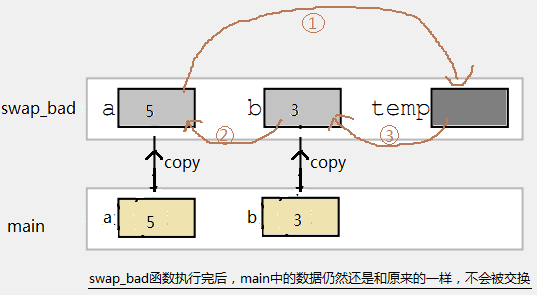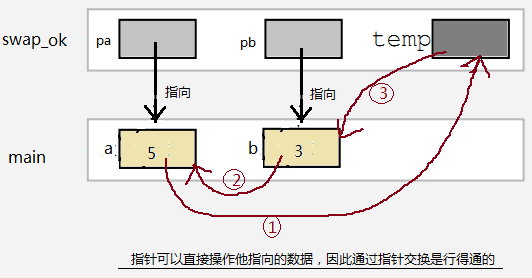``````typedef struct{
char name;
int age;
float score;
}
Student;

//打印Student变量信息
void show(const Student * ps)
{
printf("name:%s , age:%d , score:%.2f",ps->name,ps->age,ps->score);
}
``````

### 函数的指针

（关于常量指针和指针常量什么的，有时间在专门开辟一章来说明const这个东东吧，也是很有讲头的一个东东。。。）

void qsort(void*__base, size_t __nmemb, size_t __size, int(*_compar)(const void *, const void*));

``````typedef int(*compare)(const void *x, const void *y);
``````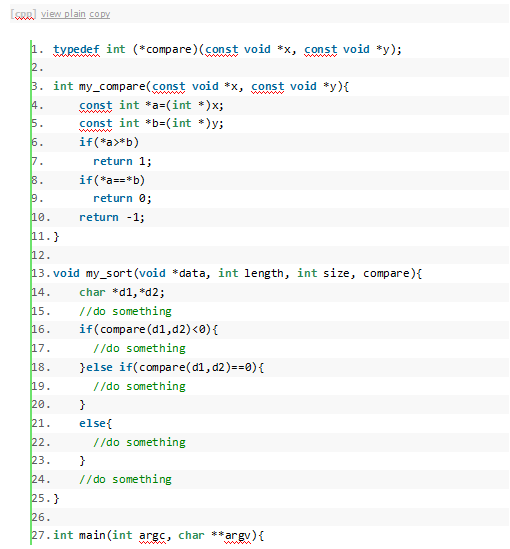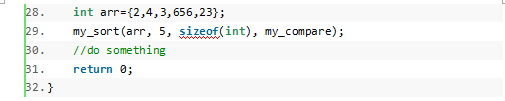C语言中，函数名作为右值时，就是这个函数的指针。

``````void echo(const char *msg)
{
printf("%s",msg);
}

int main(void){
void(*p)(const char*) = echo;   //函数指针变量指向echo这个函数
p("Hello ");      //通过函数的指针p调用函数，等价于echo("Hello ")
echo("World");
return 0;
}
``````

## 16. const和指针

const到底修饰谁？谁才是不变的？

``````int main(){
int a = 1;
int const *p1 = &a;        //const后面是*p1，实质是数据a，则修饰*p1，通过p1不能修改a的值
const int*p2 =  &a;        //const后面是int类型，则跳过int ，修饰*p2， 效果同上
int* const p3 = NULL;      //const后面是数据p3。也就是指针p3本身是const .
const int* const p4 = &a;  // 通过p4不能改变a 的值，同时p4本身也是 const
int const* const p5 = &a;  //效果同上
return 0;
} typedef int* pint_t;  //将 int* 类型 包装为 pint_t,则pint_t 现在是一个完整的原子类型

int main(){
int a  = 1;    const pint_t p1 = &a;  //同样，const跳过类型pint_t，修饰p1，指针p1本身是const
pint_t const p2 = &a;  //const 直接修饰p，同上
return 0;
}

``````

## 17. 深拷贝和浅拷贝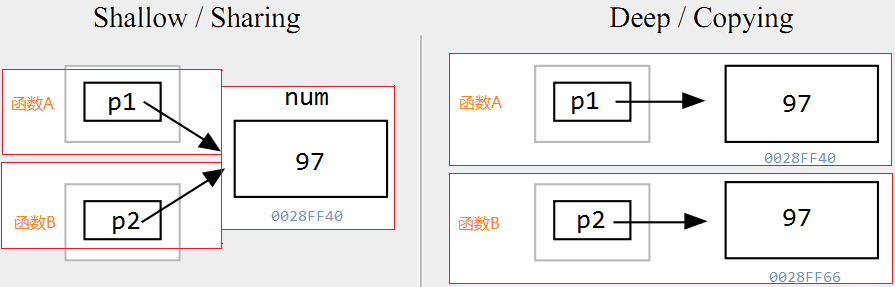## 18. 附加知识

1）Little-Endian就是低位字节排放在内存的低地址端，高位字节排放在内存的高地址端。个人PC常用，Intel X86处理器是小端模式。

1. Big-Endian就是高位字节排放在内存的低地址端，低位字节排放在内存的高地址端。

short a = 1;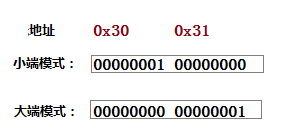//测试机器使用的是否为小端模式。是，则返回true，否则返回false
//这个方法判别的依据就是：C语言中一个对象的地址就是这个对象占用的字节中，地址值最小的那个字节的地址。

``````bool isSmallIndain()
{
unsigned int val = 'A';
unsigned char* p = (unsigned char*)&val;
//C/C++：对于多字节数据，取地址是取的数据对象的第一个字节的地址，也就是数据的低地址
return *p == 'A';
}
``````

—END—